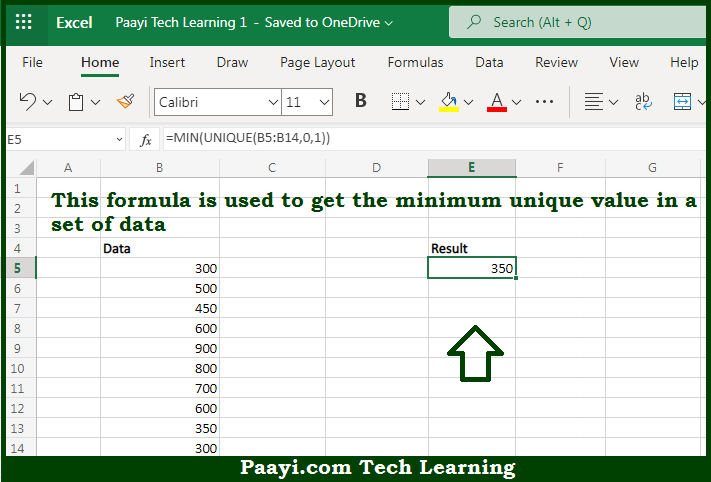# Learn How to Get Minimum Value if Unique in Microsoft Excel

Written by | 0 Comments | 495 Views

In this article, you will learn how to evaluate things in Dynamic Arrays with formulas in Microsoft Excel using a single/combination(s) of functions. You will also know How to Get Minimum Value if Unique and see the generic formula.

Learn How to Get Minimum Value if Unique in Microsoft Excel

The main purpose of this formula is to find the minimum unique value in a set of data. Here we will learn how to get the minimum value if unique in the given data range in the workbook in Microsoft Excel. That implies, with the help of a formula based on the UNIQUE, and MIN functions you can able to find the minimum unique value in a set of data. So, with the help of this formula, you can able to get a minimum value if unique in the given data range in the workbook in Microsoft Excel.

General Formula to Get Minimum Value if Unique

=MIN(UNIQUE(range,0,1))

The Explanation to Get Minimum Value if UniqueSo we know that with the help of the given formula above you can able to find the minimum unique value in a set of data. Here we will learn how to get the minimum value if unique in the given data range in the workbook in Microsoft Excel. As we know that the UNIQUE function, is new. It is used to return a unique list of values from a data set. By default, this is a list of any value that occurs one or more times in the data.

The UNIQUE function has an optional third argument called "exactly_once" that will limit results to values that occur once only in the source data. To enable this option, the argument is set to TRUE or 1. So, with the help of this formula, you can able to the minimum unique value in a set of data. So now you have learned how to get the minimum value if unique in the given data range in the workbook in Microsoft Excel.